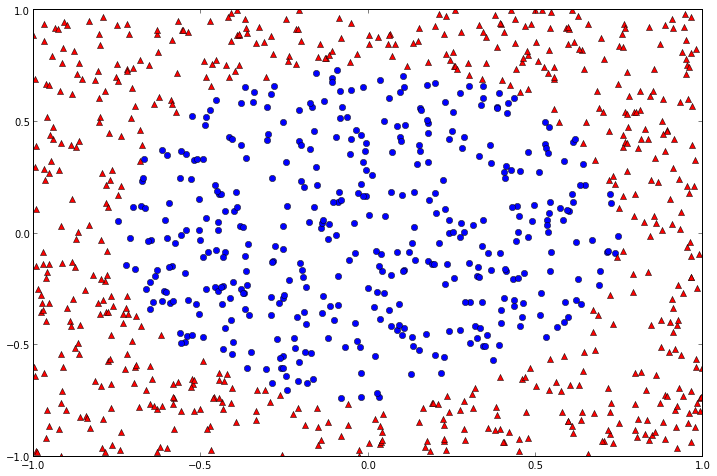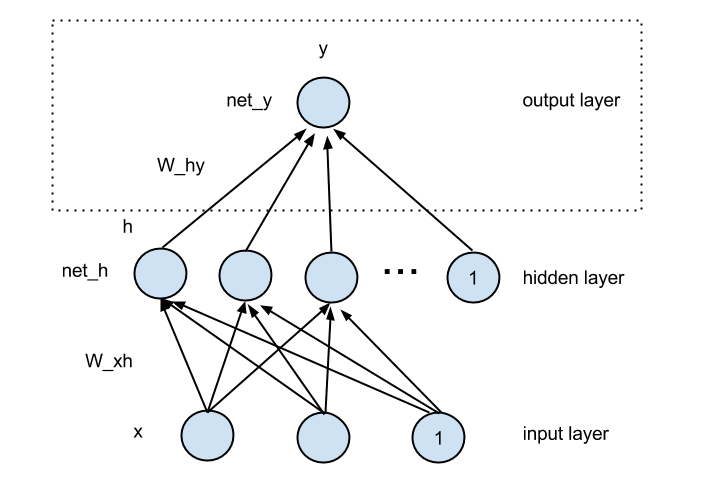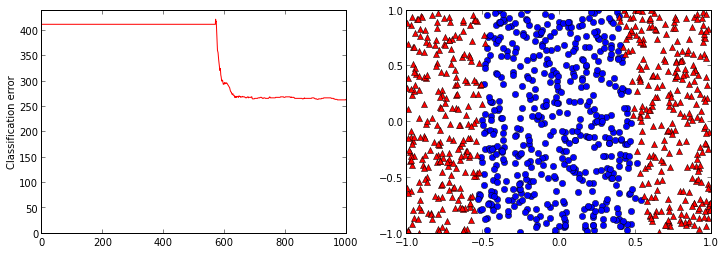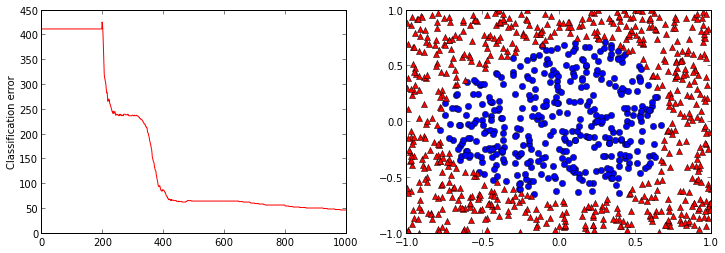# Autotelic Computing

## Wednesday, October 16, 2013

### Neural Network 101

As this seems to be becoming a series of related posts, see also:
1. Linear Regression 101
2. Logistic Regression 101
3. Softmax Regression 101
4. Neural Network 101

## A Cloud of Points¶

As powerful as it is, a logistic regression model will only ever be able to solve linearly separable problems. No need to even try on problems like the one below (even though it's quite easy), because we saw that its $\theta$ parameters correspond directly to the parameters of a linear equation in general form.

In :
rcParams['figure.figsize'] = 12, 8

def dataset(n):
xy = random.uniform(low=-1, high=1, size=(n, 2))
classes = []
for p in xy:
d = linalg.norm(p - [0,0])
classes.append(0 if d < 0.75 else 1)
return column_stack([xy, classes])

random.seed(0)
points = dataset(1000)
class0 = points[where(points[:,2] == 0)]
class1 = points[where(points[:,2] == 1)]
plot(class0[:,0], class0[:,1], 'bo')
plot(class1[:,0], class1[:,1], 'r^');## Introducing Non-Linearity¶

Although it's not 100% exact, one way to understand the classic neural network (or multilayer perceptron, as it is also sometimes called) is as an extension to the logistic regression model. If we recast our terminology in terms of neurons, layers and weights (where a neuron performs two successive computations: (1) the dot product of the incoming weights and the vector from the layer below it, and (2) the logistic squashing of the result), then LR can be seen as the top part (in the dashed rectangle) of the neural network schema shown below. The bottom part, an additional layer of weights and logistic units, is what introduces non-linearity in the model, and thus allows it to solve problems like the one above.

In :
from IPython.display import Image
Image(filename='NN.png')

Out:## Training to Get Better¶

In this simple example, since our only output neuron is logistic, we can interpret its value as a probability (of being a member of class 0 or 1), and once again endow the model with probabilistic semantics (although it is not mandatory). We can again use the negative log-likelihood as our error function

$NLL = - \sum_{i}^{n} t^{(i)} \log y^{(i)} + (1 - t^{(i)}) \log (1 - y^{(i)})$

where $y^{(i)}$ corresponds to the output of the neural network when fed with the $i$-th training example, and $t^{(i)}$ the target, its real class membership (0 or 1).

The training of a neural network is a little more involved, because the gradient of the error function needs to be back-propagated in successive computations

$\frac{\partial NLL}{\partial y} = y - t$

$\frac{\partial NLL}{\partial net_y} = y \cdot (1 - y) \cdot \frac{\partial NLL}{\partial y}$

$\frac{\partial NLL}{\partial h} = \frac{\partial NLL}{\partial net_y} \cdot W_{hy}$

$\frac{\partial NLL}{\partial W_{hy}} = \frac{\partial NLL}{\partial net_y} \cdot h$

$\frac{\partial NLL}{\partial net_y} = h \cdot (1 - h) \cdot \frac{\partial NLL}{\partial h}$

$\frac{\partial NLL}{\partial W_{xh}} = \frac{\partial NLL}{\partial net_h} \cdot x$

To finally yield the two weight updating formulas

$W_{hy} := W_{hy} - \alpha \frac{\partial NLL}{\partial W_{hy}}$

$W_{xh} := W_{xh} - \alpha \frac{\partial NLL}{\partial W_{xh}}$

In :
def logistic(x):
return 1 / (1 + exp(-x))

class NeuralNetwork:

def __init__(self, n_hiddens, alpha=0.01):
self.W_xh = random.uniform(size=(2 + 1, n_hiddens))
self.W_hy = random.uniform(size=(n_hiddens + 1, 1))
self.n_hiddens = n_hiddens
self.alpha = alpha

def train(self, x, t, n_iters=1000):
self.n = len(x)
self.x = column_stack((ones(self.n), x)) # add input bias
self.t = t
errors = []
for i in range(n_iters):
self.forward()
self.backward()
nll = -sum(self.t * log(self.y) +
(1 - self.t) * log(1 - self.y)) / self.n
classif = sum((np.round(self.y) != self.t).astype(int))
errors.append((nll, classif))
return errors

def forward(self):
self.h = column_stack((ones(self.n), # add hidden bias
logistic(dot(self.x, self.W_xh))))
self.y = logistic(dot(self.h, self.W_hy))

def backward(self):
d_nll_d_y = (self.y - self.t)
d_nll_d_net_y = self.y * (1 - self.y) * d_nll_d_y
d_nll_d_h = dot(d_nll_d_net_y, self.W_hy.T)
d_nll_d_W_hy = dot(self.h.T, d_nll_d_net_y)
d_nll_d_net_h = self.h[:,1:] * (1 - self.h[:,1:]) * d_nll_d_h[:,1:]
d_nll_d_W_xh = dot(self.x.T, d_nll_d_net_h)
self.W_hy -= self.alpha * d_nll_d_W_hy
self.W_xh -= self.alpha * d_nll_d_W_xh


The number of hidden units controls the representational power of the model. If it is too low, it will not be able to capture the complexity of the training data, as the example below shows.

In :
rcParams['figure.figsize'] = 12, 4

nn = NeuralNetwork(2)
errors = nn.train(points[:,:-1], points[:,[-1]])

_, axs = subplots(1, 2)
classif_errors = asarray(errors)[:,-1]
axs.plot(range(len(classif_errors)), classif_errors, 'r-')
axs.set_ylabel('Classification error')
axs.set_ylim(0)
points_nn = column_stack((points[:,[0,1]], np.round(nn.y)))
class0_nn = points_nn[where(points_nn[:,2] == 0)]
class1_nn = points_nn[where(points_nn[:,2] == 1)]
axs.plot(class0_nn[:,0], class0_nn[:,1], 'bo')
axs.plot(class1_nn[:,0], class1_nn[:,1], 'r^');But if it is set right, there's no limit (in theory) to the complexity of the function that the network can learn.

In :
nn = NeuralNetwork(5)
errors = nn.train(points[:,:-1], points[:,[-1]])

_, axs = subplots(1, 2)
classif_errors = asarray(errors)[:,-1]
axs.plot(range(len(classif_errors)), classif_errors, 'r-')
axs.set_ylabel('Classification error')
axs.set_ylim(0)
points_nn = column_stack((points[:,[0,1]], np.round(nn.y)))
class0_nn = points_nn[where(points_nn[:,2] == 0)]
class1_nn = points_nn[where(points_nn[:,2] == 1)]
axs.plot(class0_nn[:,0], class0_nn[:,1], 'bo')
axs.plot(class1_nn[:,0], class1_nn[:,1], 'r^');